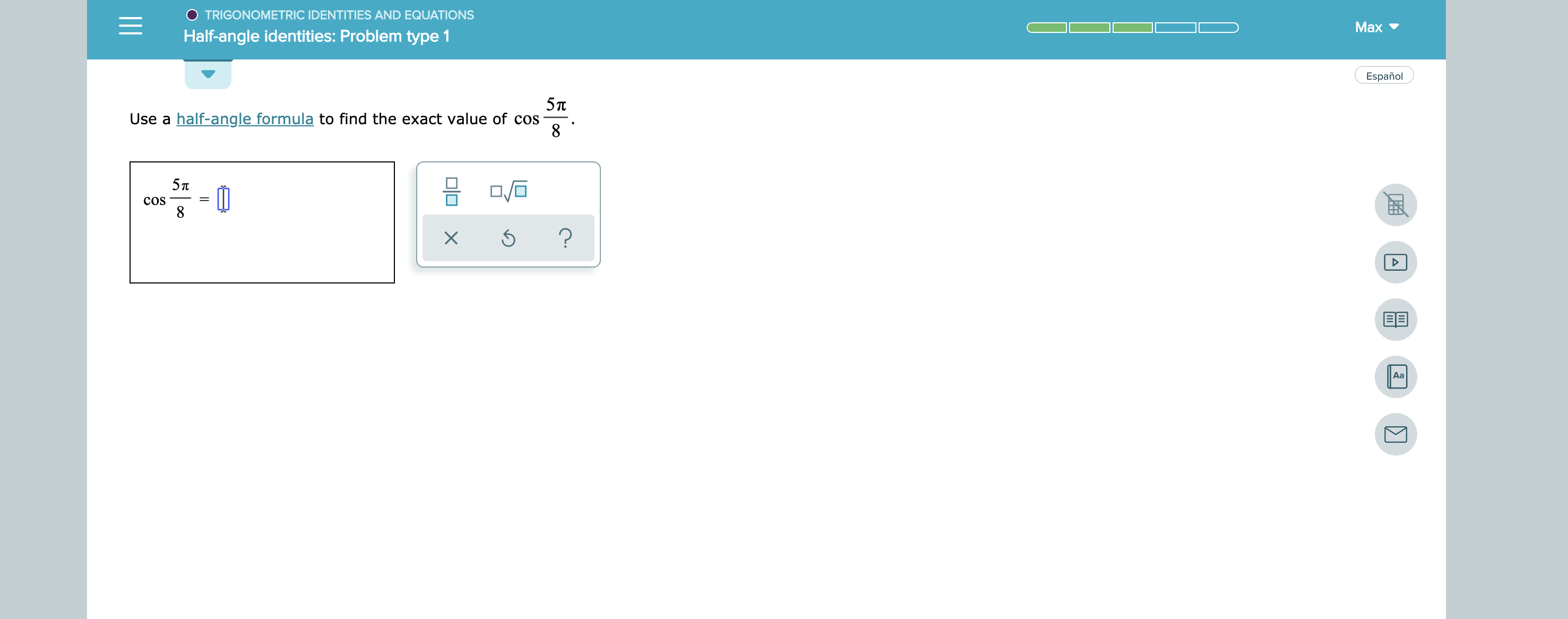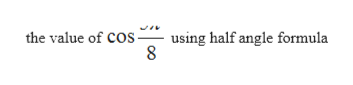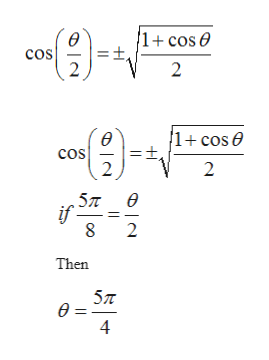TRIGONOMETRIC IDENTITIES AND EQUATIONSMaxHalf-angle identities: Problem type 1EspañolUse a half-angle formula to find the exact value of cos85пCOS8?Aa

Question

see attachmenthelp_outlineImage TranscriptioncloseTRIGONOMETRIC IDENTITIES AND EQUATIONS Max Half-angle identities: Problem type 1 Español Use a half-angle formula to find the exact value of cos 8 5п COS 8 ? Aa fullscreen
Step 1

Given function is

Step 2

To find outhelp_outlineImage Transcriptionclosethe value of COs using half angle formula 8 fullscreen
Step 3

Half angle for...help_outlineImage TranscriptioncloseCOs 2 2 1+ cos e ө cos =t, 2 2 5л е if 2 Then 5л ө- 41 fullscreen

Want to see the full answer?

See Solution

Want to see this answer and more?

Our solutions are written by experts, many with advanced degrees, and available 24/7

See Solution
Tagged in

Trigonometric Ratios